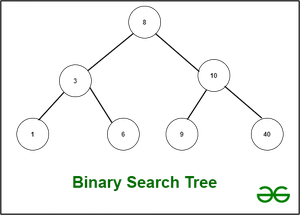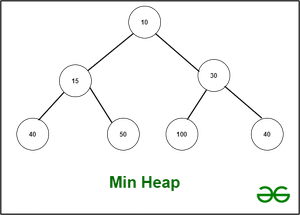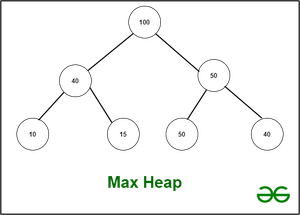# Difference between Binary Search Tree and Binary Heap

• Difficulty Level : Easy
• Last Updated : 22 Jun, 2021

1. Binary Search Tree :
An acyclic graph is commonly used to illustrate a Binary Search Tree. The tree is made up of nodes. Each node in a binary tree has no more than two children. Every node’s value is strictly higher than the value of its left child and strictly lower than the value of its right child, according to the BST. That is, we can iterate through all of the BST values in sorted order. Additionally, duplicate values are not permitted in this data structure.

There are two types of Binary Search Trees: balanced and unbalanced.

Assume that the number of nodes in a BST is n. O(n) is the worst case scenario for insert and removal operations. However, in a balanced Binary Search Tree, such as AVL or Red-Black Tree, similar operations have a time complexity of O(log(n)). Another important point to remember is that constructing a BST with n nodes takes O(n * log(n)) time. We must insert a node n times, each of which costs O(log(n)). The main benefit of a Binary Search Tree is that we can traverse it in O(n) time and receive all of our values in sorted order.2. Heap Tree :
The Heap is a Complete Binary Tree.
If the distance between a node and the root node is k, the node is at level k of the tree. The root’s level is zero. At level k, the maximum number of nodes that can exist is 2^k. A Complete Binary Tree has the maximum number of nodes at each level. Except for the last layer, which must be filled from left to right as well. It’s critical to remember that the Complete Binary Tree is always balanced.

The Heap is not the same as a Binary Search Tree. The Heap, on the other hand, is not an ordered data structure. The heap is commonly represented as an array of numbers in computer memory. It’s possible to have a Min-Heap or a Max-Heap heap. Although the features of the Min- and Max-Heaps are nearly identical, the root of the tree for the Max-Heap is the biggest number and the smallest for the Min-Heap. Similarly, the basic rule of the Max-Heap is that each node’s subtree has values that are less than or equal to the root node. The Min-Heap, on the other hand, is the polar opposite. It also implies that the Heap accepts duplicates.Binary Search Tree vs Heap :
The fundamental distinction is that whereas the Binary Search Tree does not allow duplicates, the Heap allows. The BST is ordered, while Heap is not.  So, if order is important, BST is the way to go. If an order isn’t important, but we need to know that inserting and removing data will take O(log(n)) time, the Heap assures that this will happen. If the tree is fully unbalanced, this might take up to O(n) time in a Binary Search Tree (chain is the worst case). In addition, although Heap may be built in linear time, the BST requires O(n * log(n)) to be built.

The PriorityQueue and TreeMap are Java’s implementations of these structures. By default, PriorityQueue is a Max-Heap. The backbone of TreeMap is a balanced binary search tree. A Red-Black Tree is used to implement it.

My Personal Notes arrow_drop_up